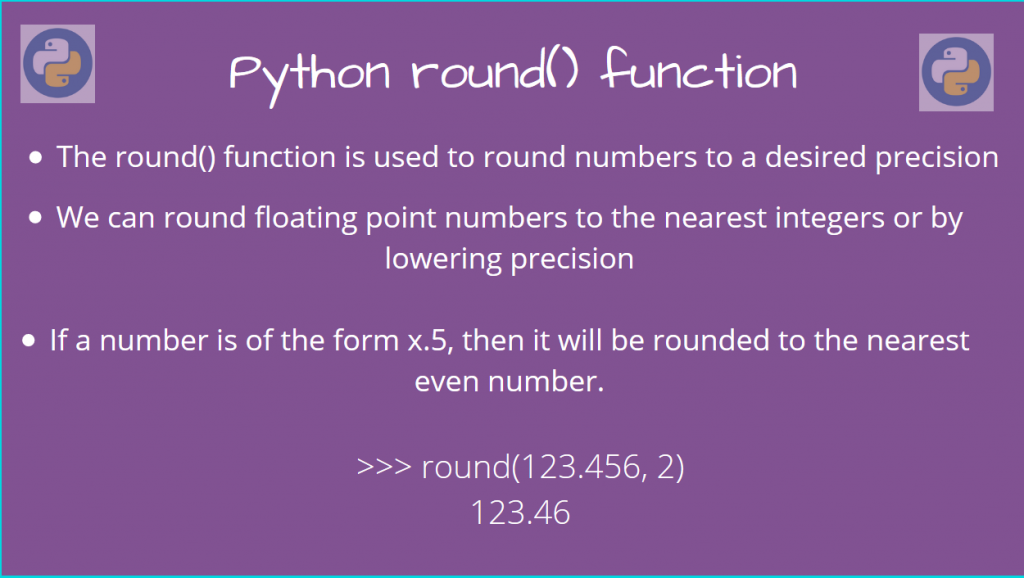# Using Python round()The Python round() function is very useful when we want to perform a rounding operation.

Often, you’d want to quickly estimate the value by rounding or reduce long floating-point decimals. round() is especially beneficial in this case.

Let’s look at how we can use it, along with some examples!

## Syntax of Python round() function

The function is very simple. It takes a number, and outputs the desired rounded number.

```round(num, [ndigits])
```

Here, we need to round `num`, so we pass it to `round()`. We can also specify the precision of the rounding using `ndigits`. This will ensure that the number will be rounded to ndigits precision after the decimal point.

If you want to round it to integer values, you don’t need to provide this value. In this case, the nearest Integer value will be returned.

Also, if the number is of the form `x.5`, then, the values will be rounded up if the roundup value is an even number. Otherwise, it will be rounded down.

For example, 2.5 will be rounded to 2, since 2 is the nearest even number, and 3.5 will be rounded to 4.

With that covered, let’s look at some examples:

## Examples of Python round()

There are some cases to consider, so let’s go about it one by one.

### 1. Integer Rounding

The below snippet shows how we can round numbers to the nearest integer.

```print(round(5, 2)) # Round 5 upto 2 decimal places. But 5 is already an integer!
print(round(6.4))
print(round(7.7))
print(round(3.5))
```

Output

```5
6
8
4
```

### 2. Rounding to even side

As mentioned earlier, if both the even and the odd sides are equally close to the number (`x.5`), then rounding will happen to the even side.

```print(round(10.5))
print(round(11.5))
```

Output

```10
12
```

### 3. Rounding with ndigit=None

If the `ndigit` argument is not specified (or `None`), rounding will happen to the nearest integer.

```print(round(2.5)) # Rounding will happen to the nearest even number
# OR
print(round(2.5, None))
```

Output

```2
2
```

### 4. Rounding with ndigit < 0

We can also pass a negative value for the `ndigit` argument. This will start rounding from the left of the decimal point!

So, if our original number has 3 digits after the decimal point, passing `ndigit = -3` will remove those 3 digits before the decimal point and replace then with 0, giving us 0!

```print(round(123.456, 0))
print(round(123.456, -1))
print(round(123.456, -2))
print(round(123.456, -3))
```

Output

```123
120.0
100.0
0.0
```

## Anomalies on rounding with floating-point numbers

Since floating-point numbers are defined by their precision, Python approximates these numbers during calculations. Due to these approximations, rounding them can give us unexpected results sometimes.

Consider the below block, where the results can be surprising:

```>>> 0.1 + 0.1 == 0.2
True
>>> 0.1 + 0.1 + 0.1 == 0.3
False
>>> 0.1 + 0.1 + 0.1 + 0.1 == 0.4
True
```

Let’s take a look at some more examples:

```>>> print(round(21.575, 2))
21.57
>>> print(round(1.23546, 2))
1.24
>>> print(round(-1.2345, 2))
-1.23
```

Again, due to the approximation of floating point, we don’t get the exact output. In the first case, we would have expected `21.58`, but we only got `21.57`.

Due to this, always be careful when dealing with floating points, as these small changes in output may give you huge problems own the line.

## Using Python round() on custom objects

The Python `round()` method internally calls the `__round__()` dunder method.

We can override this method if we’re building a custom class. Therefore, any call of `round()` on our object will go to this overriding method instead.

Let’s take a quick look at building our own custom rounder!

```class MyClass:
def __init__(self, data):
assert type(data) == type([])
self.data = data # Assume List type data of numbers
def __round__(self, num_digits=None):
for idx in range(len(self.data)):
self.data[idx] = round(self.data[idx], num_digits)
return self

my_obj = MyClass([12.34, 12.345, 10.5])
my_obj = round(my_obj)
print(my_obj.data)
```

Output

```[12, 12, 10]
```

As you can see, we were able to implement our own custom rounding function for the `MyClass` object!

## Conclusion

In this article, we learned about using the `round()` function in Python, using various examples.